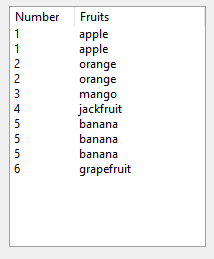# Listbox Numbering

Hi,

I want to put auto-numbering for the listbox on column 0 based from the value of column 1

``````dim i as Integer
for i = 0 to Listbox1.ListCount -1
Listbox1.Cell(i,0)=str(val(nomor.text)+1)
nomor.Text=str(val(nomor.text)+1)
next

``````

this is work, but how to keep the number is the same while column 1 has duplicate value

i am expecting the result like this, and so on depend to the listbox’s total row.any helps?

thanks
regards,
arief

try something like this:

``````
dim v as integer = 1
dim lastword as string =  Listbox1.Cell(0,1)  //first row
for i  as Integer  = 1 to Listbox1.ListCount -1
if  Listbox1.Cell(i,1)  <> lastword then
v = v +1
lastword = Listbox1.Cell(i,1)
end if
Listbox1.Cell(i,0)=str(v)
next
``````

thanks.

regards,
arief